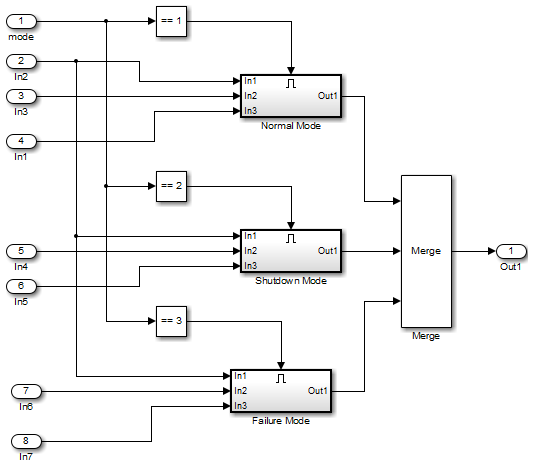Main Content

## Partition Model Inputs for Incremental Test Generation

As described in Constrain Data, you can constrain the values of model inputs using the Simulink® Design Verifier™ Test Condition block.

Like other Simulink parameters, constraint values can be shared across several blocks by referencing a common workspace variable; you can initialize constraint values using MATLAB® commands. If you have several inputs related to speed, such as desired speed, measured speed, and average speed, you might choose to constrain all of them to the same set of values.

As an advanced technique for experienced MATLAB programmers, you can use parameterized constraints and successive runs of Simulink Design Verifier to implement an incremental test-generation technique:

1. Partition model inputs so that some are held constant, some are constrained to sets of constants using the Test Condition block, and some can have any value.

2. Generate test cases and run those test cases to collect model coverage.

3. Choose new values and partition the inputs with these new values.

4. Generate test cases for missing coverage using the `sldvgencov` function and the current test coverage.

Note

To view an example of extending an existing test suite to achieve missing model coverage, enter the following at the command prompt in the MATLAB Command Window:

`showdemo('sldvdemo_incremental_test_generation')`

5. Repeat steps 3 and 4 until you have achieved the desired coverage.

Partition the model inputs that enable further simplification when an analysis runs. Consider the following model, which has three mutually independent enabled subsystems:

• Normal Mode

• Shutdown Mode

• Failure ModeYou can incrementally generate test cases for each subsystem by constraining the first input to a constant value before running an analysis. In this way, as you create test cases for each subsystem, the software ignores the complexity of the other two subsystems.

Get resources